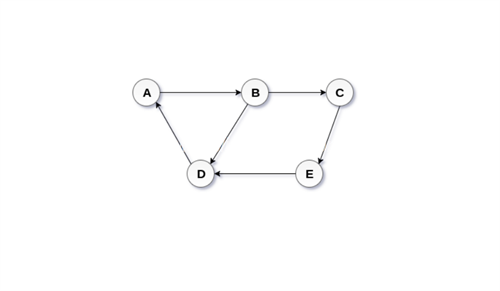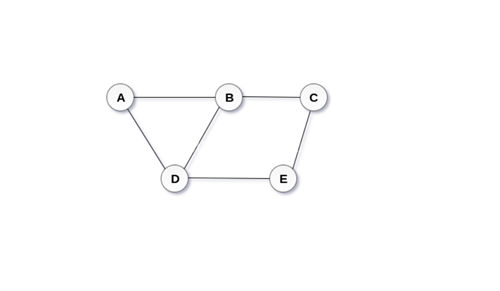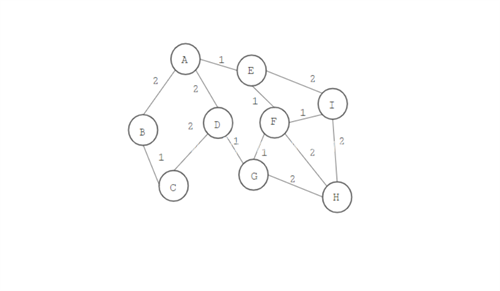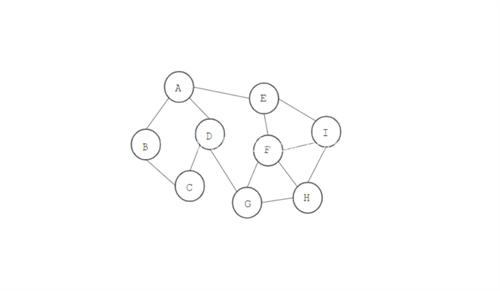Total available pages count: 55
Subject - Java Technologies

## Graph

A Graph has defined a set of vertices & edges which are used to connect such vertices. It can be visible as a cyclic tree where nodes are maintained any complex relationship among them.

Two components define it in a graph:

• A set of a number of vertices, knowns as nodes
• Edge with a finite set of ordered pair in for (u,v)

## Classification

Graph the non-linear data structure, classified on bases of two-parameter: Direction & Weight.

## Direction

Direction classified as directed graph & undirected graph.

## Directed graph

A directed graph is a collection of nodes or vertices that are connected with each other & all edges have direction from one vertex to another.## Undirected graph

An undirected graph is a group of node or vertices which are connected to each other with no direction## Weight

The graph's non-linear data structure can be classified as a weighted graph & an unweighted graph.

## Weighted graph

A graph in which weight can be present on every edge of the graph. a weighted graph is a type of labeled graph.## Unweighted graph

A graph in which no weight present on any edge.